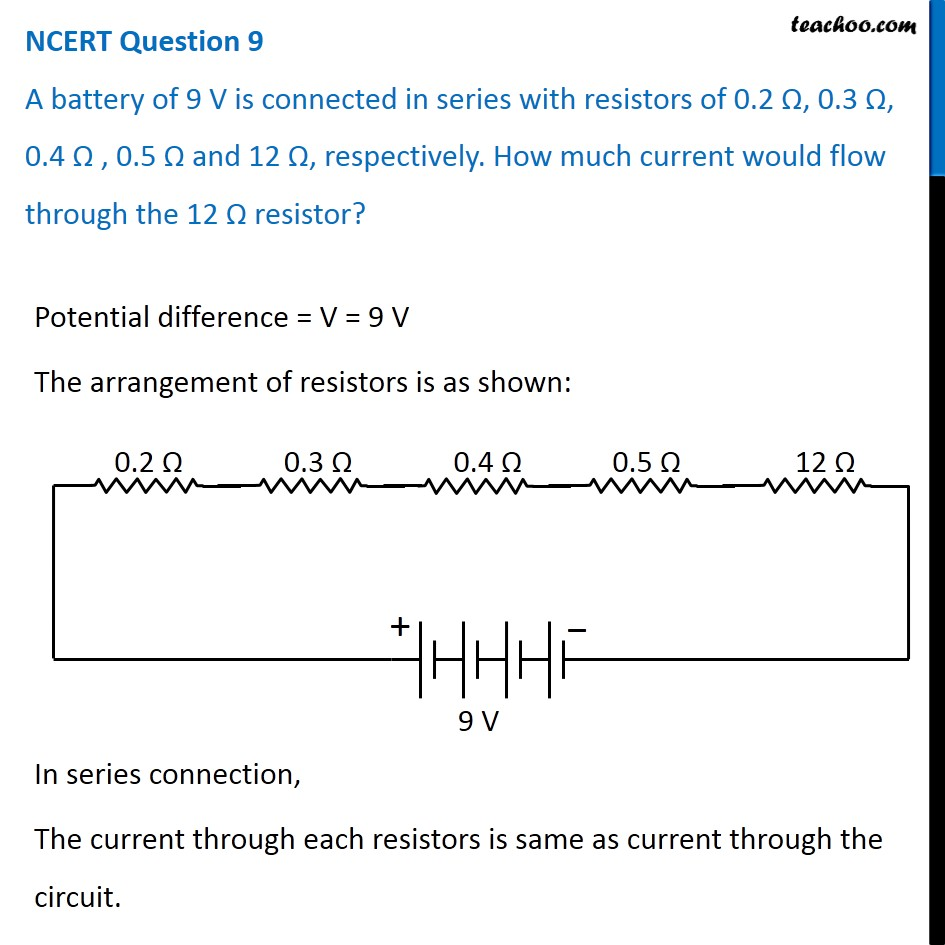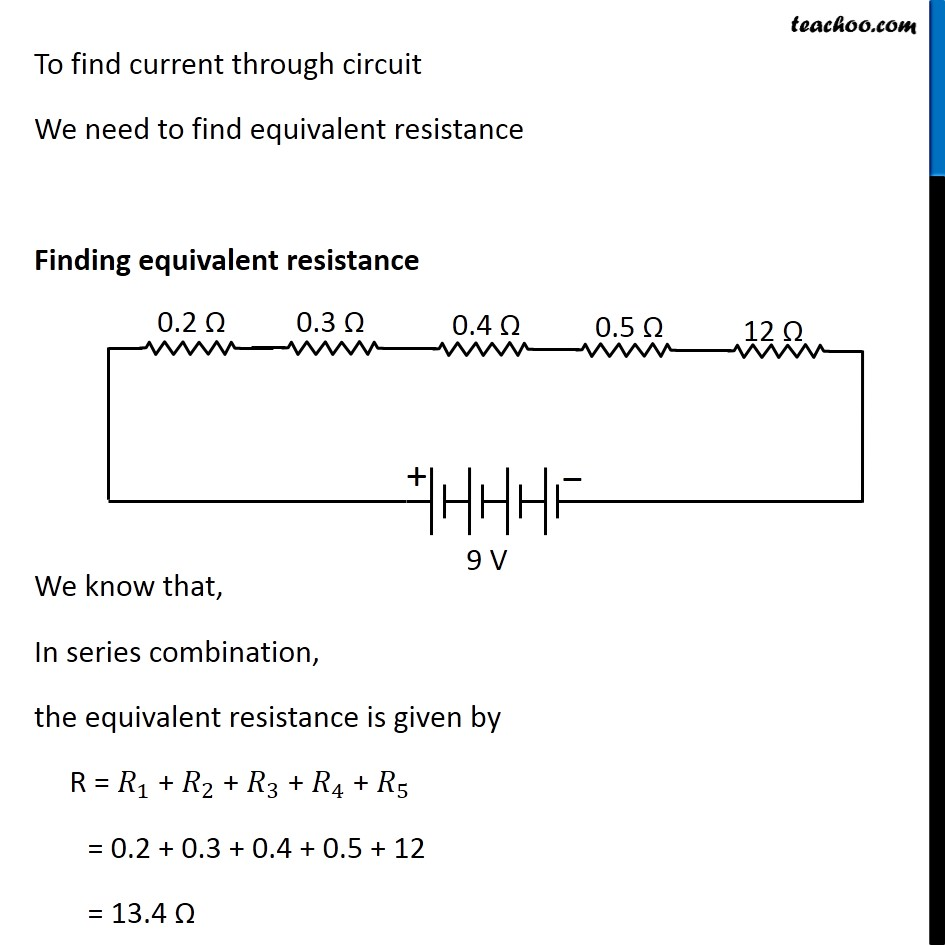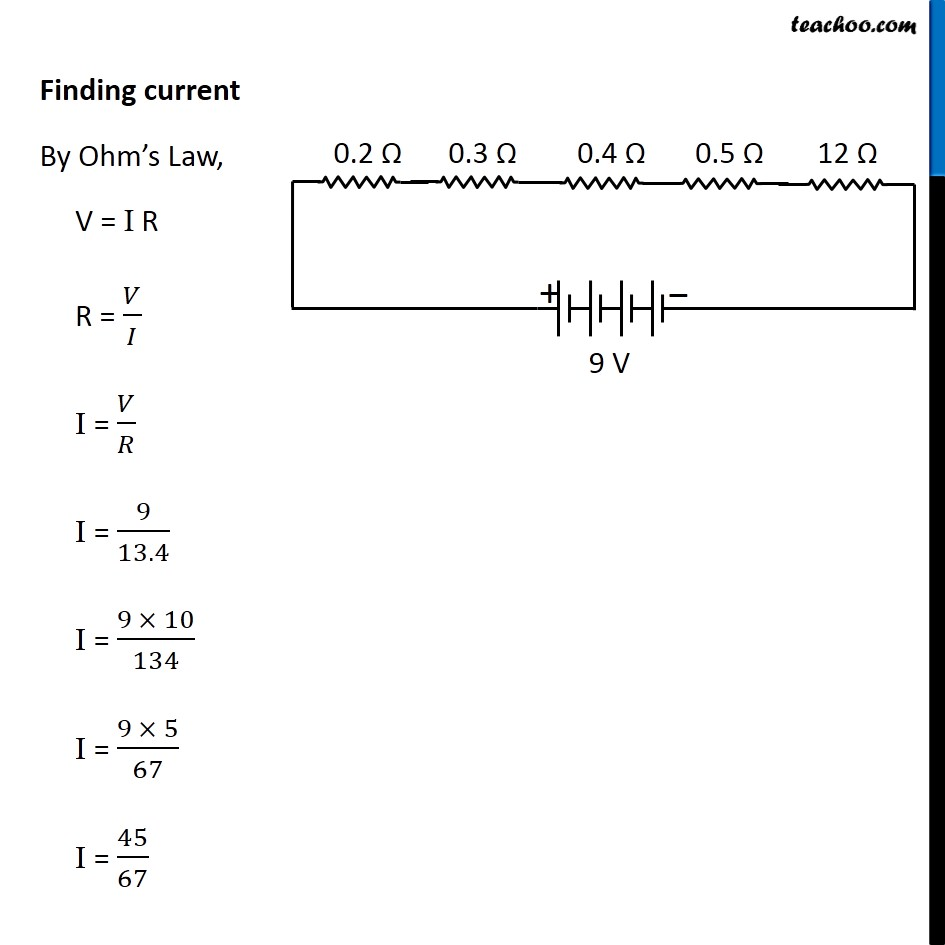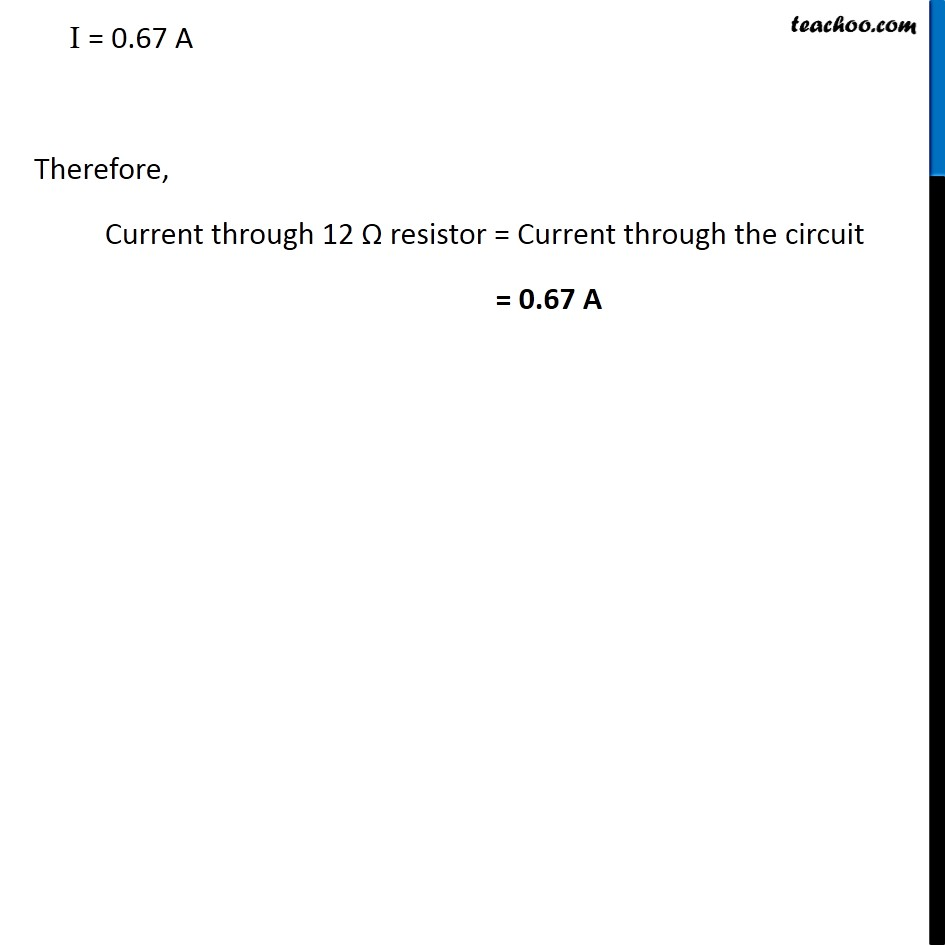NCERT Questions

Class 10
Chapter 12 Class 10 - Electricity

## A battery of 9 V is connected in series with resistors of 0.2 Ω, 0.3 Ω, 0.4 Ω , 0.5 Ω and 12 Ω, respectively. How much current would flow through the 12 Ω resistor?Learn in your speed, with individual attention - Teachoo Maths 1-on-1 Class

### Transcript

NCERT Question 9 A battery of 9 V is connected in series with resistors of 0.2 Ω, 0.3 Ω, 0.4 Ω , 0.5 Ω and 12 Ω, respectively. How much current would flow through the 12 Ω resistor? Potential difference = V = 9 V The arrangement of resistors is as shown: In series connection, The current through each resistors is same as current through the circuit. To find current through circuit We need to find equivalent resistance Finding equivalent resistance We know that, In series combination, the equivalent resistance is given by R = 𝑅_1 + 𝑅_2 + 𝑅_3 + 𝑅_4 + 𝑅_5 = 0.2 + 0.3 + 0.4 + 0.5 + 12 = 13.4 Ω Finding current By Ohm’s Law, V = I R R = 𝑉/𝐼 I = 𝑉/𝑅 I = 9/13.4 I = (9 × 10)/134 I = (9 × 5)/67 I = 45/67 I = 0.67 A Therefore, Current through 12 Ω resistor = Current through the circuit = 0.67 A